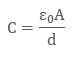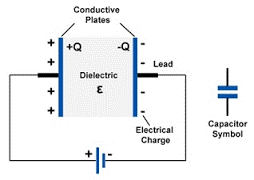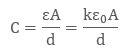# Notes on capacitance of a parallel plate capacitor with and without dielectric medium between the plates.

2 years ago

## Capacitance of Parallel Plate Capacitor

The capacitance of parallel plate capacitor depends upon:

• The distance d between two plates

• The area A of medium between the plates

• According to the gauss law, the electric field can be written asSince we know that the capacitance is defined as V = Q/C, so capacitance can be rewritten asWhen the plates areplaced very close and the area of plates are large we get the maximum capacitance.

## Capacitance of Parallel Plate Capacitor when dielectric slab is placedFig 1.7 dielectric placed between two electrodes

On the two plates, the microscopic dipole moment of the material will shield the charges. Thus, will alter the effect of dielectric material which is inserted between the two plates Materials have a permeability which is given by the relative permeability K

The capacitance is thus given by:Capacitance of a parallel plate capacitor can be increased by introducing dielectric between the plates as the dielectric have permeability k, which is greater than 1. K is also sometimes known as Dielectric Constant.